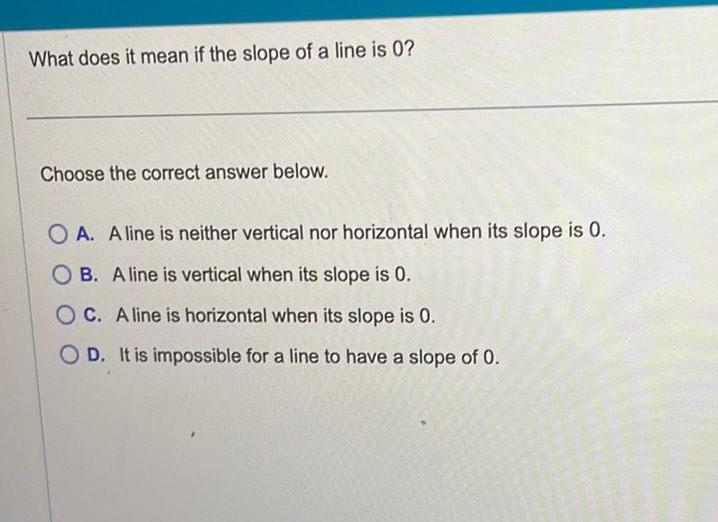Question:

# What does it mean if the slope of a line is 0 Choose the

Last updated: 9/18/2023What does it mean if the slope of a line is 0 Choose the correct answer below OA A line is neither vertical nor horizontal when its slope is 0 OB A line is vertical when its slope is 0 OC A line is horizontal when its slope is 0 OD It is impossible for a line to have a slope of 0